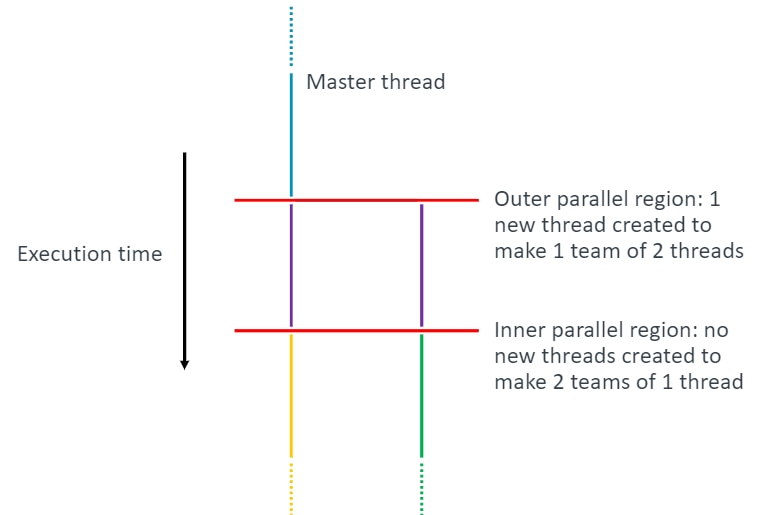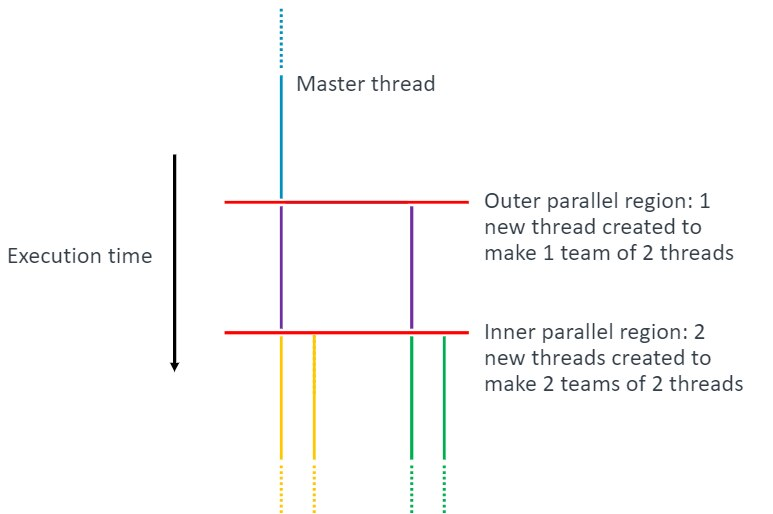## OpenMP thread mapping

#### Open MP settings

Overview Setting the number of threads Control the placement of threads Report OpenMP settings Related information

## Set the number of OpenMP threads

To set the number of threads to use in your program, set the environment variable `OMP_NUM_THREADS`. `OMP_NUM_THREADS` sets the number of threads used in OpenMP parallel regions defined in your own code, and within Arm Performance Libraries. If you set `OMP_NUM_THREADS` to a single value, your program uses a single level of parallelism. In this case, nested parallelism is disabled.

Note: The information about setting `OMP_NUM_THREADS` applies to both compilers supported by Arm Performance Libraries in release 21.0: Arm Compiler 21.0 and GCC 10.2.

For example, consider the following code, which defines a nested parallel region:

```#include <stdio.h>
#include <omp.h>

int main() {
#pragma omp parallel
{
printf("outer: omp_get_thread_num = %d omp_get_level = %d\n", omp_get_thread_num(), omp_get_level());
#pragma omp parallel
{
printf("inner: omp_get_thread_num = %d omp_get_level = %d\n", omp_get_thread_num(), omp_get_level());
}
}
}

> armclang -o a1.out -fopenmp threading.c
> OMP_NUM_THREADS=2 ./a1.out
outer: omp_get_thread_num = 0 omp_get_level = 1
inner: omp_get_thread_num = 0 omp_get_level = 2
outer: omp_get_thread_num = 1 omp_get_level = 1
inner: omp_get_thread_num = 0 omp_get_level = 2

> gcc -o g1.out -fopenmp threading.c
> OMP_NUM_THREADS=2 ./g1.out
outer: omp_get_thread_num = 0 omp_get_level = 1
inner: omp_get_thread_num = 0 omp_get_level = 2
outer: omp_get_thread_num = 1 omp_get_level = 1
inner: omp_get_thread_num = 0 omp_get_level = 2
```

The program above reports the thread number and level of parallel nesting. Executables built with either GCC or Arm Compiler for Linux show the same behavior when `OMP_NUM_THREADS` is set to a single value (and all other settings use default values).

The example above sets `OMP_NUM_THREADS=2` and the output shows that two threads are used for the outer parallel region. The nested parallel regions create no new threads :Note: The actual number of threads used during execution of your program might differ from the value specified in `OMP_NUM_THREADS` if the number of threads is set explicitly in the code using the OpenMP API, or if a system-defined limit is encountered.

`OMP_NUM_THREADS` can also be set to a comma-separated list of values. Where a list of values are passed to `OMP_NUM_THREADS`, the values denote the number of threads to use at each level of nesting, starting from the outermost parallel region.

The default behavior when using a list of values with `OMP_NUM_THREADS` differs between Arm Compiler for Linux and GCC. For example, using the same executables as compiled earlier:

```> OMP_NUM_THREADS=2,2 ./a1.out

outer: omp_get_thread_num = 0 omp_get_level = 1
outer: omp_get_thread_num = 1 omp_get_level = 1
inner: omp_get_thread_num = 0 omp_get_level = 2
inner: omp_get_thread_num = 1 omp_get_level = 2
inner: omp_get_thread_num = 0 omp_get_level = 2
inner: omp_get_thread_num = 1 omp_get_level = 2

> OMP_NUM_THREADS=2,2 ./g1.out
outer: omp_get_thread_num = 0 omp_get_level = 1
inner: omp_get_thread_num = 0 omp_get_level = 2
outer: omp_get_thread_num = 1 omp_get_level = 1
inner: omp_get_thread_num = 0 omp_get_level = 2 ```

The example above specifies that the two parallel regions in the code can each use two threads. The Arm-compiled executable creates a new thread in each of the two inner parallel regions, enabling nested parallelism:However, the GCC-compiled executable shows the same output as with `OMP_NUM_THREADS=2`, keeping nested parallelism disabled.

The reason for this difference in behavior is because the OpenMP runtime provided with Arm Compiler for Linux version 21.0 uses `OMP_NESTED=true` when `OMP_NUM_THREADS` is a comma-separated list. The OpenMP runtime provided with the GCC 10.2 compiler has `OMP_NESTED=false` when `OMP_NUM_THREADS` is a comma-separated list.

Notes:

• The `OMP_NESTED` setting is being deprecated for OpenMP 5.0.
• This is a change of behavior for executables linked to the OpenMP runtime in Arm Compiler for Linux version 21.0. Previous Arm Compiler for Linux behavior matched the current behavior for gcc.

To enable nested parallelism for the GCC-compiled executable, explicitly turn on nesting:

```> OMP_NESTED=true OMP_NUM_THREADS=2,2 ./g1.out
outer: omp_get_thread_num = 0 omp_get_level = 1
outer: omp_get_thread_num = 1 omp_get_level = 1
inner: omp_get_thread_num = 0 omp_get_level = 2
inner: omp_get_thread_num = 1 omp_get_level = 2
inner: omp_get_thread_num = 0 omp_get_level = 2
inner: omp_get_thread_num = 1 omp_get_level = 2
```

Nested parallelism in Arm Performance Libraries is handled in the same way as shown in these examples; if an Arm Performance Libraries routine is called from a parallel region in your code, then the routine spawns threads in the same way as shown for the nested parallel region in the examples above.